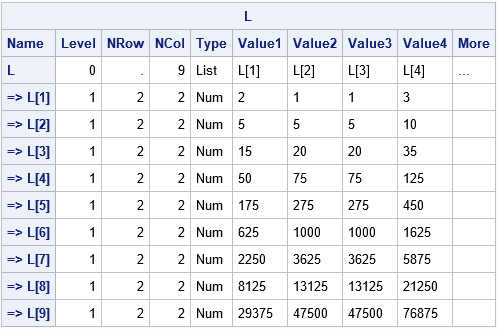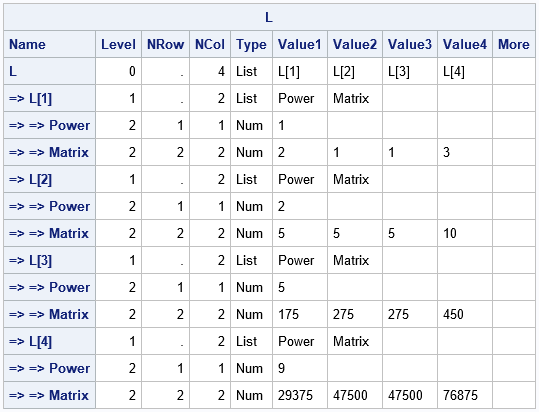Suppose that a data set contains a set of parameter values. For each row of parameters, you need to perform some computation. A recent discussion on the SAS Support Communities mentions an important point: if there are duplicate rows in the data, a program might repeat the same computation several times. This is inefficient. An efficient alternative is to perform the computations once and store them in a list. This article describes this issue and implements it by using lists in SAS/IML software. Lists were introduced in SAS/IML 14.2 (SAS 9.4M4). A natural syntax for creating lists was introduced in SAS/IML 14.3 (SAS 9.4M5).

This article assumes that the matrices are not known until you read a data set that contains the parameters. The program must be able to handle computing, storing, and accessing an arbitrary number of matrices: 4, 10, or even 100. Obviously, if you know in advance the number of matrices that you need, then you can just create those matrices and give them names such as X1, X2, X3, and X4.

### Example: Using powers of a matrix

To give a concrete example, suppose the following data set contains multiple values for a parameter, p:

```data Powers; input p @@; datalines; 9 1 5 2 2 5 9 1 2 5 2 1 ;```

For each value of p, you need to compute X = Ap = A*A*...*A for a matrix, A, and then use X in a subsequent computation. Here's how you might perform that computation in a straightforward way in SAS/IML without using lists:

```proc iml; /* use a small matrix, but in a real application the matrix might be large. */ A = {2 1, 1 3};   use Powers; /* read the parameters from the data set */ read all var "p"; close;   /* The naive method: Compute the power A**p[i] each time you need it. */ do i = 1 to nrow(p); power = p[i]; X = A**power; /* compute each power as needed */ /* compute with X ... */ end;```

The program computes Ap for each value of p in the data set. Because the data set contains duplicate values of p, the program computes A2 four times, A5 three times, and A9 two times. If A is a large matrix, it is more efficient to compute and store these matrices and reuse them as needed.

### Store all possible matrices in a list

If the values of p are uniformly likely, the easiest way to store matrix powers in a list is to find the largest value of p (call it m) and then create a list that has m items. The first item in the list is A, the second item is A2, and so forth up to Am. This is implemented by using the following statements:

```/* Method 1: Find upper bound of power, m. Compute all matrices A^p for 1 <= p <= m. Store these matrices in a list so you don't need to recompute. */ m = max(p); /* largest power in data */ L = ListCreate( m ); do i = 1 to m; L\$i = A**i; /* compute all powers up to maximum; store A^i as i_th item */ end;   do i = 1 to nrow(p); /* extract and use the matrices, as needed */ power = p[i]; X = L\$power; /* or X = ListGetItem(L, power); */ /* compute with X ... */ end;```

For these data, the list contains nine elements because 9 is the largest value in the data. The following statements show how to display a compact version of the list, which shows the matrices Ap for p=1,2,...,9.

```package load ListUtil; /* load the Struct and ListPrint subroutines */ run struct(L);```The table shows the first few elements of every item in the list. In this case, the p_th item is storing the 2 x 2 matrix Ap. The elements of the matrix are displayed in row-major order.

### Store only the necessary matrices in a list

Notice that the data only contain four values of p: 1, 2, 5, and 9. The method in the previous section computes and stores unnecessary matrices such as A3, A4, and A8. This is inefficient. Depending on the maximum value of p (such as max(p)=100), this method might be wasteful in terms of memory and computational resources.

A better solution is to compute and store only the matrices that are needed for the computation. For this example, you only need to compute and store four matrices. You can use the UNIQUE function to find the unique values of p (in sorted order). The following statements compute and store Ap for only the unique values of p in the data set:

```/* Method 2: Compute matrices A^p for the unique values of p in the data. Store only these matrices in a named list. */ u = unique(p); /* find the unique values of p */ nu = ncol(u); /* how many unique values? */ L = ListCreate( nu ); do i = 1 to nu; power = u[i]; /* compute only the powers in the data; store in a named list */ L\$i = [#"Power" = power, /* optional: store as a named list */ #"Matrix" = A**power]; end;   run struct(L);```For this example, each item in the list L is itself a list. I used a "named list" with items named "Power" and "Matrix" so that the items in L have context. The STRUCT subroutine shows that L contains only four items.

How can you access the matrix for, say, A5? There are several ways, but the easiest is to use the u matrix that contains the unique values of p in sorted order. You can use the LOC function to look up the index of the item that you want. For example, A5 is stored as the third item in the list because 5 is the third element of u. Because each item in L is itself a list, you can extract the "Matrix" item as follows:

```do i = 1 to nrow(p); power = p[i]; k = loc(u = power); /* the k_th item is A^p */ X = L\$k\$"Matrix"; /* extract it */ /* compute with X ... */ *print i power X; end;```

In summary, this article describes how to use a list to store matrices so that you can compute them once and reuse them many times. The article assumes that the number of matrices is not known in advance but is obtained by reading a data set at run time. The example stores powers of a matrix, but this technique is generally applicable.

If you have never used lists in SAS/IML, see the article "Create lists by using a natural syntax in SAS/IML" or watch this video that describes the list syntax.

Share